元气骑士武器怎么合成-元气骑士合成表大全

最近有很多萌新玩家表示还不太清楚元气骑士武器怎么合成?那么接下来了小编就为的玩家们带来了元气骑士武器合成表大全，希望可以帮助到各位玩家哦。

强力分裂者+绿色响尾蛇=蝰蛇(攻击后子弹爆开附带跟踪效果带暴击毒伤)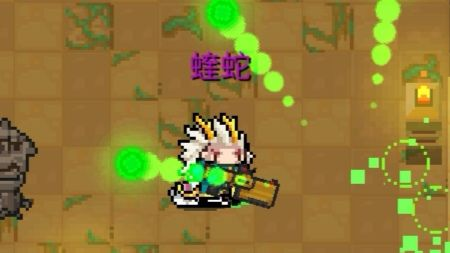光剑红+光剑蓝=光剑紫(可以普攻可以蓄力，蓄力后进行扇形平移扫射)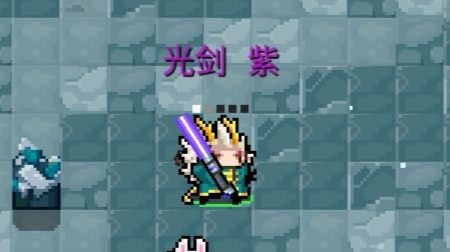火箭筒(不是火箭炮)+冰川=冰爆(射出后炸裂出一块冰屑，并在地上残留一段时间)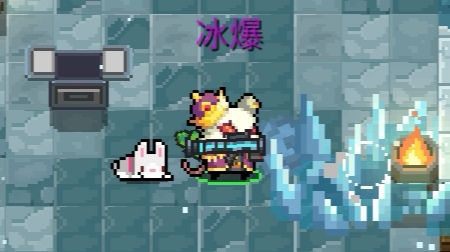大雪狐+弩=灰狐(双联发弩)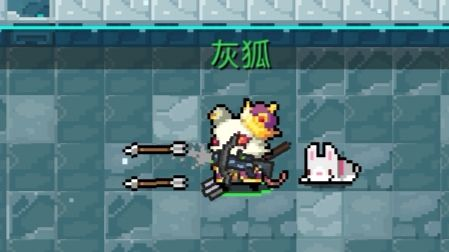外星之鹰+粒子之鹰=等离子之鹰(弹射类大光球暴击附带闪电标记，再攻击附带伤害)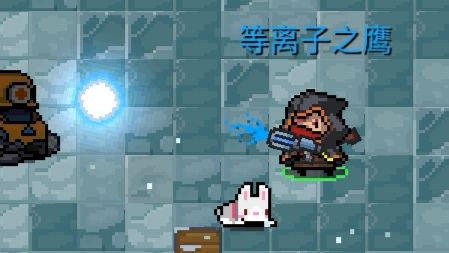1、雪狐系列

雪狐+雪狐=大雪狐

大雪狐+大雪狐=巨雪狐

巨雪狐+巨雪狐=巨巨雪狐

雪狐土豪金+樱花霰弹枪=雪狐玫瑰金

2、时代系列

欠欠时代冲锋枪+欠欠时代冲锋枪=欠时代时代冲锋枪

欠时代冲锋枪+欠时代冲锋枪=次时代冲锋枪

次时代冲锋枪+次时代冲锋枪=次次时代冲锋枪

二次时代冲锋枪+二次时代冲锋枪=三次时代冲锋枪

三次时代冲锋枪+三次时代冲锋枪=四次时代冲锋枪

3、黄金喷漆

狙击步枪+黄金喷漆=黄金狙击步枪

左轮+黄金喷漆=黄金左轮

精致法杖+黄金喷漆=黄金精致法杖

法杖+黄金喷漆=黄金法杖

沙漠之鹰+黄金喷漆=黄金沙漠之鹰

阿卡47+黄金喷漆=黄金阿卡47

4、蓝色喷漆

加特林+蓝色喷漆=蓝火加特林

织网者=蓝色喷漆=剧毒织网者

5、进阶套件

法杖+进阶套件=精致法杖

雪狐+进阶套件=大雪狐

大雪狐+进阶套件=巨雪狐

巨雪狐+进阶套件=巨巨雪狐

巨雪狐+进阶套件=雪狐土豪金

雪狐土豪金+进阶套件=雪狐玫瑰金

欠欠时代冲锋枪+进阶套件=欠时代冲锋枪

欠时代冲锋枪+进阶套件=次时代冲锋枪

次时代冲锋枪+进阶套件=次次时代冲锋枪

次次时代冲锋枪+进阶套件=次次次时代冲锋枪

次次次时代冲锋枪+进阶套件=次次次时代冲锋枪

冲锋枪一代+进阶套件冲锋枪二代

冲锋枪二代+进阶套件=冲锋枪三代

冲锋枪三代+进阶套件=冲锋枪四代

霞弹枪一代+进阶套件=霞弹枪二代

霞弹枪二代+进阶套件=霞弹枪三代

初始武器+进阶套件=初始武器进阶版

离子轨道炮+进阶套件=离子轨道炮二代

守卫者轨道炮+进阶套件=电磁轨道炮

黄金狙击步枪+进阶套件=黄金狙击枪专业版

雷电战锤+进阶套件=雷暴战锤

武士刀+进阶套件=40米长刀

短截散弹枪+进阶套件=超级散弹枪

光之杖+进阶套件=女神锡杖

攻城弩+进阶套件=床子弩

6、便携式烤炉

大葱+便携式烤炉=烤大葱

萝卜+便携式烤炉=烤萝卜

肉+便携式烤炉=烤肉

鱼+便携式烤炉=烤鱼

尚方宝剑+便携式烤炉=烤尚方宝剑

激光鱼+便携式烤炉=烤激光鱼

7、时间机器

双刃剑+时间机器=光剑红

武士刀+时间机器=光剑蓝

改良冲锋枪+时间机器=次时代冲锋枪

破旧的手枪+时间机崭新的手枪

老旧的狙击枪+时间机器=狙击步枪

老旧的火箭筒+时间机器=火箭筒

老旧的卡宾枪+时间机器=美国卡宾枪

大巫师的旧法杖+时间机器=大巫师之杖

8、种田

萝卜成熟后再生长一次=百年萝卜

竹子成熟后再生长一次=千年笋

9、手枪

两把不同應(雪人不算)+樱花霞弹枪=喂嘤鷹

冰霜之鹰+火焰之鹰=冰与火之鹰

p250+p250=双管手枪

破旧的手枪4=妙妙枪

10、步枪

杀猪刀+狙击步枪=刺枪

杀猪刀+老旧的狙击枪=刺枪

杀猪刀+黄金狙击步枪=刺枪

杀猪刀+黄金狙击枪专业版=刺枪

熔炉+冰川=极光

冲锋枪一代+冲锋枪一代=螺旋冲锋枪

冲锋枪三代+冲锋枪三代=冲锋枪四代

突击步枪+突击步枪=强化突击步枪

强化突击步枪+强化突击步枪=强化突击步枪

强化突击步枪+仲裁者=突击步枪战术精英版(雷电)

强化突击步枪+审判者=突击步枪战术精英版(眩晕)

强化突击步枪+碎冰者=突击步枪战术精英版(冰冻)

强化突击步枪+可恶激光枪=突击步枪战术精英版(剧毒)

强化突击步枪+火箭炮=榴弹机枪

11、霞弹

红黄蓝冲锋枪+骑士之拳=彩虹

骑士之拳+闪电法杖=天堂之拳

彩虹+加特林=彩虹加特林

红色响尾蛇绿色响尾蛇=沙罗曼蛇

12、导弹

喇叭+火箭炮=聚爆

13、激光

网瘾少年+网瘾少女=羊叫兽

审判者+审判者=散射激光枪

14、弓望

英雄弓+风之弓=魔法弓

火焰弓+瑶之弓+冰霜弓=群星之弓

弓/强弓+弓/强弓=巨弓

督+弩=攻城弩

攻城弩+TNT=爆炸弩

弩+TNT=爆炸弩

15、法杖

大葱+木棍=自然法杖

大葱+浪潮法杖=有机后浪

16、近战

战斧+火之杖=火焰战斧

木棍+斧子=长柄斧

火焰剑+冰霜剑=雷剑

光剑红光剑蓝+光剑绿=光剑土豪金

破碎的剑柄+破碎的剑刀=重铸的圣剑

浪潮法杖+干草叉=三叉戟

仲裁者+标枪=激光枪

锤子+TNT=爆炸战锤

17、投掷

冰锥+标枪=冰枪

手里剑+手里剑=风魔手里剑

风魔手里剑+风魔手里剑=风之手里剑

电弧手里剑+电弧手里剑=量子手里剑

雷电战锤+电弧手里剑=宙斯之矛

千本+外星之鹰=千本喂(眩晕)

千本+剧毒之鹰=千本喂(剧毒)

千本+火焰之鹰=干本喂(灼烧)

千本+冰霜之鹰=千本喂(冰冻)

干本+粒子之鹰=干本喂(雷电)

18、生活

塞子+塞子=拔粪宝

无尽能量药水+无尽生命药水=无尽恢复药水

鱼+碎冰者=激光鱼

四种特殊合成机制介绍

第一种

武器+喷漆配件=新武器

第二种

食品武器+便携式烤炉配件=熟了的可食用武器

第四种

种在花圃内的成熟植物+反复浇水=进阶植物

以上就是小编带来的元气骑士武器合成表大全，感兴趣的朋友不要错过哦。

热门推荐

• 末日来袭

版本： 安卓版 v1.2.2大小：639.15MB

末日来袭安卓版是一款末日丧尸题材的冒险生存游戏，末日来袭游戏中会有很多的丧尸入侵你们的家园，你要要消灭...

• 翡翠帝国特别版

版本： 1.0.0.143255大小：3.61 GB

《翡翠帝国特别版》是由AspyrMedia,Inc.出品的一款角色扮演游戏，游戏开始时，玩家可选择7名各具特色的游戏...

• 剑侠世界3

版本： 安卓版 v1.6.5711大小：1.76GB

剑侠世界3安卓版是一款由西山居全新推出的武侠对战类手游。剑侠世界3最新版游戏采用了精致唯美的画风打造...

• 逗斗火柴人

版本： 安卓版 v4.4.0.68704大小：532.17MB

逗斗火柴人安卓版是一款由Steam平台《StickFight：The Game》正版授权而来的多人竞技类手游。在逗斗火柴...

• 幻塔

版本： 安卓版 v1.2.78.41297大小：1.72GB

幻塔安卓版是一款高度自由的开放世界角色扮演类手游。幻塔最新版手游采用虚幻4引擎打造，动作捕捉系统赋予...